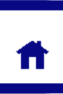# Molar Heat of Combustion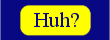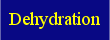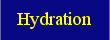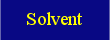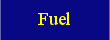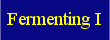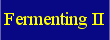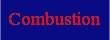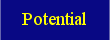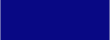Students learn to define the molar heat of combustion of a compound and calculate the value for ethanol from first-hand data

We have already said that burning one mole of ethanol releases 1409.4 kilojoules of heat. This value - the amount of heat generated by the complete combustion of one mole of a substance - is called the molar heat of combustion.

The molar heat of combustion is the amount of heat released by the complete combustion (reaction with oxygen gas to form water and carbon dioxide) of one mole of a substance. The molar heat of combustion is expressed in J/mol (or kJ/mol).

One way the molar heat of combustion may be determined experimentally is by calorimetry. From the word calorimetry you may have already worked out what the experiment may entail. Basically, you burn a known amount of the substance in plenty of oxygen and see what the temperature change of a known body is.

If we were to do a simple calorimetric experiment, we might use the following simple apparatus: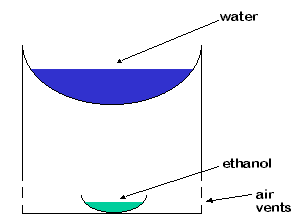In the experiment, we add a small amount of ethanol and burn it, measuring the starting temperature and maximum temperature reached by the water using a thermometer. From these data, we can work out how much energy was released, hence estimate the heat of combustion for the ethanol.

Let's look at some typical results for such an experiment...